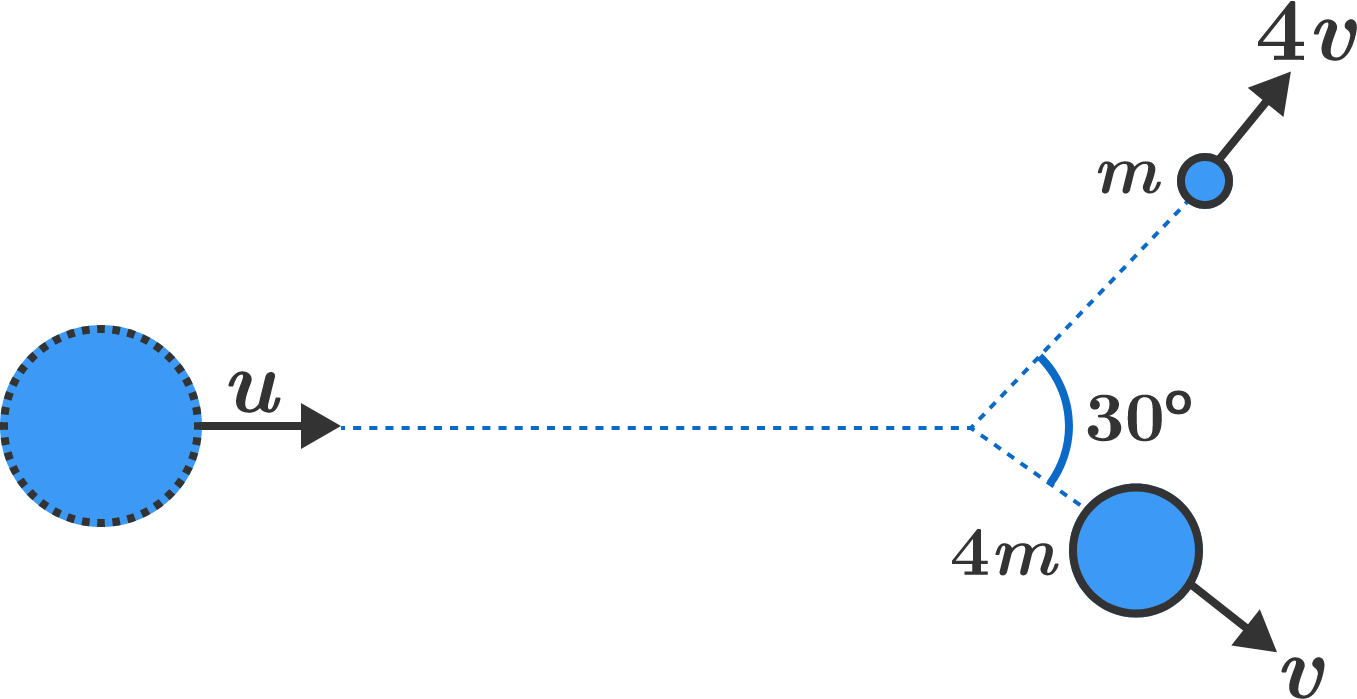# How fast was the parent?

Two particles of masses $m$ and $4m$ have velocities of $4v$ and $v,$ respectively. They are moving at an angle of $30^\circ$ with respect to each other.

If the particles have come from a decay of a single parent particle, and both mass and momentum are conserved, how fast was the parent particle moving?Note: For the purposes of this problem, ignore energy conservation requirements and relativistic effects.

×